• 검색 결과가 없습니다.

# Development of Quasi-Optical Millimeter- and THz-wave System for Probing Plasma Density

N/A
N/A
Protected

Share "Development of Quasi-Optical Millimeter- and THz-wave System for Probing Plasma Density "

Copied!
74
0
0
더 보기 ( 페이지)

전체 글

In addition, we show that this ICP chamber can be used for a plasma switching system for the study of high power millimeter wave in pulsed plasma. The developed ICP chamber was used for fast control technique of high power millimeter wave using pulsed plasma. For heat testing, a gyrotron at UNIST (95 GHz, 20 kW power) is used as a high power millimeter wave source.

## Introduction

### Overview of millimeter- and THz-waves in free space and complex dielectric media

Nevertheless, measuring conductive media in quasi-optical systems is still not common, despite quasi-optics having an advantage as a measurement system. For this reason we present the developed measurement technique of complex permittivity in conductive media such as semiconductors and plasma. Based on these techniques, we have also developed their applications such as semiconductor lifetime measurement for defect observation and plasma diagnostics of high-density inductively coupled plasma (ICP).

### Thesis outline

Furthermore, we have proposed a suitable modeling of the plasma density and carrier density in semiconductors within a quasi-optical millimeter and THz wave system. In addition, this thesis will discuss rapid control of high-power millimeter waves using pulsed plasma.

## Concept of quasi-optical transmission system in millimeter- and THz-waves

### Low loss quasi-optical transmission system

• Gaussian beam using corrugated horn antenna
• Quasi-optical mirror with mode matching technique
• Oversized corrugated waveguide for HE11 mode transmission

For practical situations that have axial symmetry (e.g., wave horn antenna, lenses, and mirrors), we can express the axisymmetric paraxial wave equation (6) from (5). Therefore, the Gaussian beam can be transmitted to the target point using dielectric lenses and metal mirrors. The advantage of a quasi-optical mirror is that we can plan the beam propagation path with the desired size and position of the beam using geometrical optics.

### Theory of complex permittivity measurement using quasi-optical technique

1+𝜌122𝜌232𝑒−4𝑣2𝜂+2𝜌12𝜌23𝑒−2𝑣2𝜂cos[𝜙12+𝜙23+2𝑢2𝜂] (36) indexing and ref. decay function, we can obtain time-transient transmittance and reflectance of TE wave in a semiconductor. The large-scale plasma thickness can be modeled as a multilayer plasma and the EM wave propagation can be calculated using a propagation matrix method . When the plasma layer comprises several layers, starting from l = 1 to l = N, and the non-plasma layer is the free space at l = 0 and l = N + 1, the boundary conditions can be represented by

In the (𝑙 + 1)th region, we can express the propagation matrix, 𝑉̅𝑁𝑃, for the internal propagation in the same layer. 50) Finally, the derived matrix of columns in the (N+1)th region can be reorganized as

## Measurement of the carrier lifetime in semi-conductor using a quasi-optical millimeter and

### Concept of carrier lifetime measurement in semi-conductor

Second, the penetration of the microwave signal decreases in a thick silicon wafer (> 100 µm) in the case of a high doping density (> 1015 cm−3). In the microwave region, the reflectance of the Si sample increases with doping density, which leads to poor sensitivity with a thick sample as shown in Figure 3-3. Therefore, detecting a change in reflectance in a transient manner using the existing μ-PCD technique is difficult.

The µ-PCD method can measure a semiconductor with a low injection level and is used under small disturbances (Δσ ≪ 𝜎0) -. For low purity and low crystalline quality Si, the estimation of such wafers using low injection conditions on which the µ-PCD method is based is usually invalid due to the low signal to noise ratio and misreading of the measured lifetime out of its working condition . The measurement data is thus distorted, which can cause errors in the analysis of the decay time of the photoconductivity.

In this study, we developed a technique called quasi-optical photoconductor decay (QO-PCD) for measuring sample lifetime using an antenna and receiver in the millimeter and terahertz (THz) wave regime . In these regimes, covering frequencies between 100 GHz and 1 THz, there is a window for minimizing the reflectivity of thick samples or high doping densities. The reflectance above 100 GHz may be available for measuring samples with high doped density, because the reflectance is low enough to ensure high sensitivity measurements compared to the reflectance in the microwave regime in µ-PCD –.

Furthermore, a Gaussian beam can be sent to a receiver with a beam waist size that can be reduced by increasing the frequency in the case of a quasi-optical system.

### Quasi-optical photoconductivity decay (QO-PCD) method

• Theory of photoconductivity decay
• Experimental setup of quasi-optical system

Based on the theory, we can simply simulate QO-PCD system as shown in Figure 3-6. In the experimental setup, a 95 GHz Vector Network Analyzer (VNA, N5247A, Keysight Technologies) was used as a millimeter wave source with an amplifier (DET-10-RPNW1, Gain: 14 dB, Millitech Inc.). The beam size of optical pump laser is approx. 1.57mm in full width at half maximum (FWHM).

The Gaussian beam was transferred from a corrugated feed horn to a wafer through a quasi-optical focusing mirror. The quasi-optical off-axis ellipsoidal (QO-OAE) mirror reflects the beam propagation direction by 90°. Furthermore, the phase of the waist is almost flat in the sample position and the receiver position as shown in Figure 3-9 and Figure 3-10.

Before the carrier lifetime was measured, the initial information for the Si sample was needed. This quasi-optical transmission system can measure the dielectric constant, thickness and initial carrier density (dark conductivity) of Si sample by using frequency sweep in VNA. Finally, we can measure S21 parameter for Si in quasi-optical system as shown in figure 3-13.

The results agree well with the theoretical model with a convergence error of about 1% based on Eq.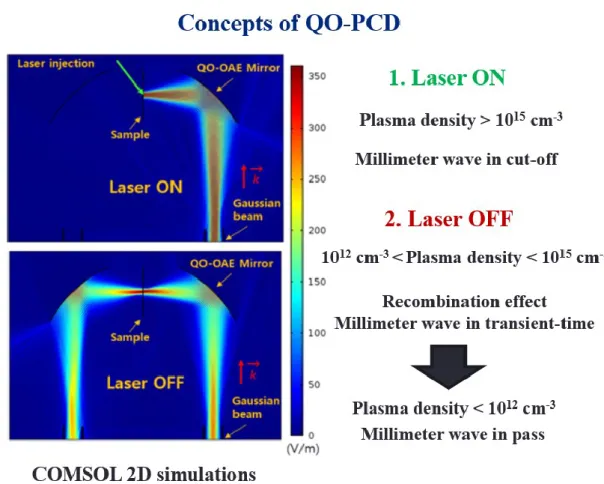Figure 3-6. The path of gaussian beam with and without photo-excitations in 2D simulation (COMSOL Multiphysics)

### Experimental study of QO-PCD system

The error due to the non-ideal linear fitting curve shown in Figure 3-16 (b) was less than 10%. The important feature of the QO-PCD method is that the area under measurement can be focused to a small spot, which prevents the interference of unnecessary areas. The QO-PCD technique has been proven to measure a wide range of photoconductivity decay time changes because the high sensitivity contributes to the high-frequency measurement system, as described in Figure 3-16. RF transmittance signal with optical pumping compared to the theoretical model.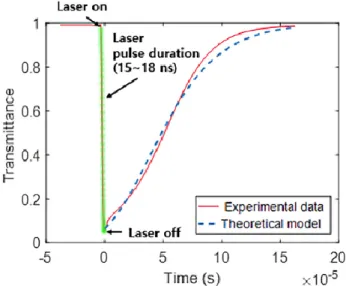Figure 3-15. RF transmittance signal with optical pumping compared to the theoretical model

## Characteristics of high-power millimeter-wave in pulsed plasma

Motivation

### Design of inductive rf discharged high-density plasma

The characteristic impedance of the fabricated ICP chamber was measured by VNA and this result is in good agreement with the theoretical calculation in Figure 4-2.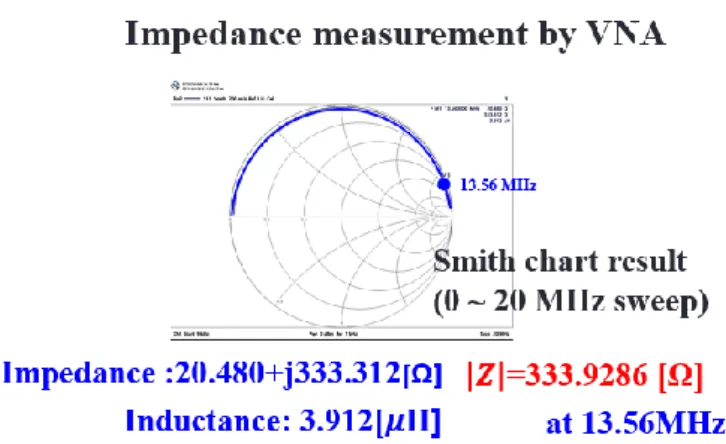Figure 4-2. Impedance measurement of ICP chamber (Frequency sweep: 0 ~ 20 MHz)

### Experimental setup of Plasma switching system

• Experimental setup
• Analysis of pulsed plasma using non-invasive millimeter-wave
• Axisymmetric two-dimensional multi-layer model for plasma sheath

Given the dominance of bulk ohmic heating in high-density plasma, we can assume that the plasma density is distributed along an axis only along the propagation direction of the EM wave. To estimate the propagation of the EM waves in the ICP plasma, we model the cosine distribution of the plasma density to multilayer isotropic media . Therefore, the cylindrical coordinate with its origin at the center of the plasma, the plasma density profile, 𝑛(𝑟), in the radial direction, can be expressed as.

Finally, we can derive the function of plasma density comprising a radially flat-topped distribution function and a longitudinal cosine distribution function. For Gaussian beam propagation in plasma, the transmittance in the 2D multilayer plasma is not uniform in the radial position, as shown in Figure 4-6. In a flat top model, the radial plasma density is higher than the Bessel model plasma density as shown in Figure 4-8(a).

On the other hand, increasing the Ar gas pressure results in an increase in the plasma density and a decrease in the effective collision frequency. The first behavior follows the existing results of ICP plasma experiments and the second behavior follows the report of G. Lister within the Maxwellian distribution. a) Comparison of plasma density distributions between radial plasma models (r-axis, flat-top and Bessel models) (b). Using plasma sheath analysis, we can estimate the effective thickness of the plasma sheath by assuming that the plasma density in the sheath is defined by Eq.

Considering Figure 4.11, we can estimate that the effective thickness of the plasma sheath decreases as the plasma density increases.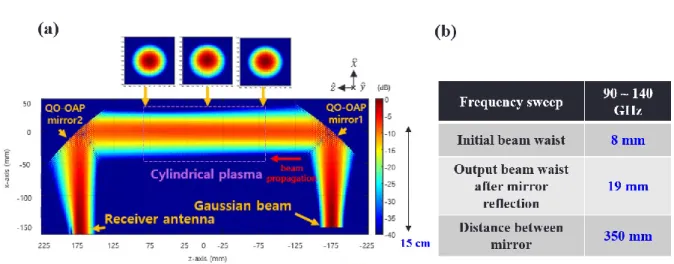Figure 4-3. (a) Simulation results of QO-OAP mirrors using Surf3D code (b) Specifications of Gaussian beam in QO system

### Fast control of high-power millimeter pulse in pulsed plasma

• Gyrotron: high-power millimeter wave source
• Focusing mirror
• Experimental setup
• Absorption of high-power millimeter wave in steady-state plasma
• High-power millimeter-wave in pulsed plasma for plasma switching system

Experimental Setup of High Power Millimeter Wave Absorption in Pulsed Plasma Gaussian Beam Focused in Plasma Chamber with Engineered Focusing Mirror Grid. Schottky diode detectors measure high power millimeter wave transmittance and reflection after the plasma absorption effect. In this setup, we perform experiments to identify high-power millimeter wave absorption in plasma.

When steady-state plasma is generated, a high-power millimeter wave is launched into the plasma. In the other hands, we can find transmittance and reflectance of high-power millimeter wave using Schottky diode detector in experiment. The optical emission signal increases suddenly with the ratio of high power millimeter wave signal as described in Figure 4-22(c).

Based on the results of high-power millimeter waves in steady-state plasma, we attempted to extend high-power millimeter waves in pulsed plasma for high-speed switching application of high-power millimeter waves using plasma switching system. We need to check the low-power millimeter-wave interaction in pulsed plasma to understand the physical effects before performing a high-power millimeter-wave test. If we assume the CW of high power millimeter wave, the absorbed millimeter wave will increase the plasma density and maintain the boundary plasma density at section 4.

Understanding the plasma physics in high-power millimeter-wave absorption pulsed plasma is very challenging because the plasma density and effective collision frequency change periodically, but high-power millimeter-wave absorption also has effects of plasma parameter change.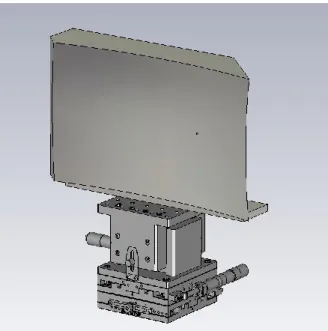Figure 4-14. CST MWS simulation of reflection gain measurement in focusing mirror

## Conclusion

In high-power millimeter-wave section, we performed experiments on high-power millimeter-wave absorption in steady-state plasma and pulsating plasma. In the experiment, high-power millimeter wave can be absorbed in steady-state plasma without high reflection. On the other hand, pulsed plasma can pass high power millimeter wave in plasma 'OFF' time.

Choi, “Carrier lifetime measurement using millimeter and THz wave quasi-optical system,” Appl. Theurer, “High-speed switching of far-infrared radiation by photoionization in a semiconductor,” Appl Opt, vol. Katayama, “Terahertz dielectric response of photoexcited carriers in Si revealed by direct optical pump and terahertz probe spectroscopy,” Appl.

Neilson, “Surf3d and LOT: Computer Codes for Design and Analysis of High-Performance QO Launchers in Gyrotrons,” Infrared Millim. Bunger, “Surface integral equation analysis of quasi-optical launch vehicles using the fast multipole method,” Conf. Suk, “Characterization of the electron density of inductively coupled argon plasmas by the terahertz time domain spectroscopy,” Plasma Sources Sci.

You et al., “Measurement and analysis of the electron-neutral collision frequency in the calibrated boundary probe,” Phys.

수치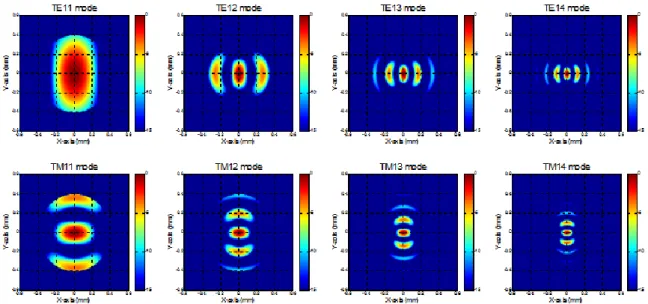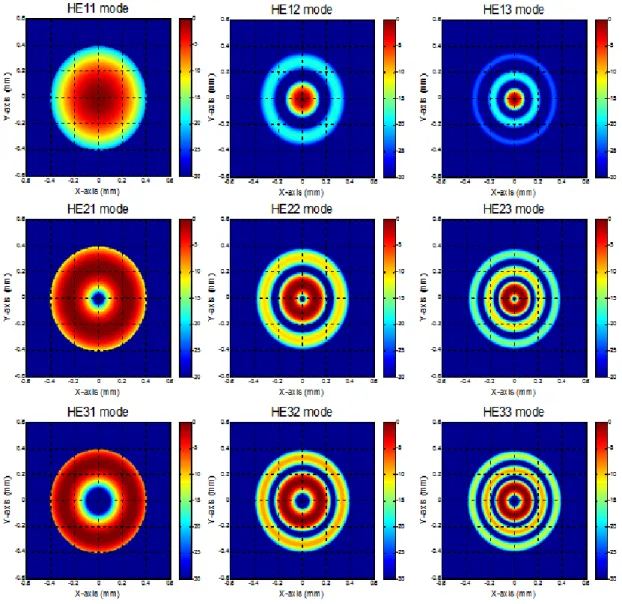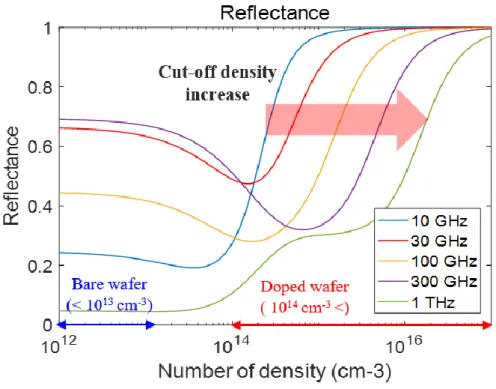+7

참조

관련 문서

선교활동 의 경우 현지인들에 의해 문제가 제기되면 어려움을 겪게 되므로 현지 문화를 존중하는 범위 내에서 선교활동을 해야 하며 특히, 종 교 비자 없이 임의로 선교활동

우리는 이전에 구한 타이틀을 이용하여 남자의 평균, 기혼.. feature vector

of PDLSCC), there was a significant correlation (p&lt;0.05) of β-catenin aberrant expression and the histological degrees , whereas in normal epithelia The staining

In the ultrasonographic images, the appearance for abnor- mal spleen could be roughly divided into 5 types: 1) splenom- egaly with normal echotexture; 2) diffuse parenchymal

Although several studies have reported that photofunctionalization improves the wettability and bioactivity of titanium surfaces, they have mostly examined

programming skills, outstanding research potential in machine learning (e.g, recurrent networks, reinforcement learning,.. evolution, statistical

2.9절에서 연립 미분방정식으로 표시되는 몇 가지 간단한 모델에 대해

The agreement could open up new channels of cooperation with a more moderate Iran and help stabilize the Middle East, or it could cause increased destabiliz- ing competition

reconciliation between the Afghan government and the Taliban, the next administration should take a look at what more can be done to re-energize whole of government ‘ways’ to

언어 선택하기

웹사이트는 선택한 언어로 번역됩니다.

기타 언어